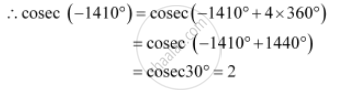CBSE (Arts) Class 11CBSE
Share

# Find the Value of the Trigonometric Function Cosec (–1410°) - CBSE (Arts) Class 11 - Mathematics

ConceptSigns of Trigonometric Functions

#### Question

Find the value of the trigonometric function cosec (–1410°)

#### Solution

It is known that the values of cosec repeat after an interval of 2π or 360°.Is there an error in this question or solution?

#### APPEARS IN

NCERT Solution for Mathematics Textbook for Class 11 (2018 to Current)
Chapter 3: Trigonometric Functions
Q: 7 | Page no. 63

#### Video TutorialsVIEW ALL 

Solution Find the Value of the Trigonometric Function Cosec (–1410°) Concept: Signs of Trigonometric Functions.
S